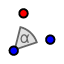# Lesson 3.5 Rotations Investigation

Topic:
Rotation

## DIRECTIONS:

In the GeoGebra applet below: 1) Create a slider that is an angle. Set Min = 0 degrees. Set Max = 360 degrees. Set Increment = 1 degree. 2) Choose the ROTATE AROUND POINTtool. Highlight a box around point A, point B, and Daffy Duck's pic. Select point C as the point about which to rotate the items you've just selected. For angle, go to the menu on the right and choose . 3) Select the MOVE tool. 4) Move the slider right and left. Note the images of points A and B (denoted as A' and B'). Feel free to move points A and B around as well. After doing all this, please answer the questions that appear below the applet.

## 1.

Let C = (0,0) be the point about which points A and B (and Daffy Duck) are rotated. Place point A at (2, 3) and point B at (5, 1). Set . What are the coordinates (x, y) of the image of A? What are the coordinates (x, y) of the image of B?

## 2.

Let C = (0,0) be the point about which points A and B (and Daffy Duck) are rotated. Place point A at (2, 3) and point B at (5, 1). Set What are the coordinates (x, y) of the image of A? What are the coordinates (x, y) of the image of B?

## 3.

Let C = (0,0) be the point about which points A and B (and Daffy Duck) are rotated. Place point A at (2, 3) and point B at (5, 1). Set . What are the coordinates (x, y) of the image of A? What are the coordinates (x, y) of the image of B?

## 4.

Let (0,0) be the point about which points A and B (and Daffy Duck) are rotated. Suppose the coordinates of point A are now labeled as (x, y). Now even though we don't know what the coordinates of point A are, can you write expressions (in terms of x and/or y) for the coordinates of the image of A under a a) 90 degree counterclockwise rotation about (0,0)? b) 180 degree counterclockwise rotation about (0,0)? c) 270 degree counterclockwise rotation about (0,0)?